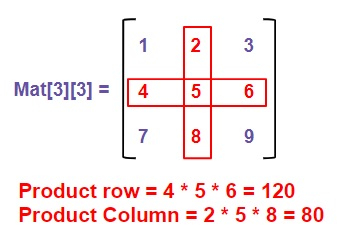# Product of middle row and column in an odd square matrix in C

Given a square matrix, mat[row][column] where row and column are equal and are of odd length means the number of row and column must me odd, i.e, not divisible by 2, the task is to find the product of middle row and middle column of that matrix.

Like in the given figure below −## Constraints

• Matrix must be a square matrix.

• Column and rows must be of odd length.

Input

mat[][] = {{1, 2, 3},
{4, 5, 6},
{7, 8, 9}}

Output

Product of middle row = 120
Product of middle column = 80

Explanation

Product of middle row = 4 * 5 * 6 = 120
Product of middle column = 2 * 5 * 8 = 80

Input

mat[][] = {{3, 5, 0},
{1, 2, 7},
{9, 0, 5}}

Output

Product of middle row = 14
Product of middle column = 0

Explanation

Product of middle row = 1 * 2 * 7 = 120
Product of middle column = 5 * 2 * 0 = 0

## Approach used below is as follows to solve the problem

• Take a matrix mat[][] as an input.

• Traverse the matrix as from the middle row and middle column

• Calculate the product of the middle row and middle column and return the result.

## Algorithm

Start
In function int product(int mat[][MAX], int n)
Step 1→ Declare and initialize rproduct = 1, cproduct = 1
Step 2→ Loop For i = 0 and i < n and i++
Set rproduct = rproduct * mat[n / 2][i]
Set cproduct = cproduct * mat[i][n / 2]
Step 3→ Print "Product of middle row: rproduct “
Step 4→ Print "Product of middle column: cproduct”
In function int main()
Step 1→ Declare and initialize mat[][MAX] {
{ 1, 2, 3 },
{ 4, 5, 6 },
{ 7, 8, 9 } }
Step 2→ Call product(mat, MAX)
Stop

## Example

Live Demo

#include <stdio.h>
#define MAX 3
int product(int mat[][MAX], int n){
int rproduct = 1, cproduct = 1;
//We will only check the middle elements and
//find their products
for (int i = 0; i < n; i++) {
rproduct *= mat[n / 2][i];
cproduct *= mat[i][n / 2];
}
// Printing the result
printf("Product of middle row: %d", rproduct);
printf("Product of middle column: %d", cproduct);
return 0;
}
// Driver code
int main(){
int mat[][MAX] = {
{ 1, 2, 3 },
{ 4, 5, 6 },
{ 7, 8, 9 } };
product(mat, MAX);
return 0;
}

## Output

If run the above code it will generate the following output −

Product of middle row: 120
Product of middle column: 80